x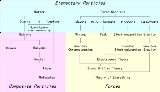Bound stateEncyclopedia
In physics
Physics
Physics is a natural science that involves the study of matter and its motion through spacetime, along with related concepts such as energy and force. More broadly, it is the general analysis of nature, conducted in order to understand how the universe behaves.Physics is one of the oldest academic...

, a bound state describes a system where a particle
Particle
A particle is, generally, a small localized object to which can be ascribed physical properties. It may also refer to:In chemistry:* Colloidal particle, part of a one-phase system of two or more components where the particles aren't individually visible.In physics:* Subatomic particle, which may be...

is subject to a potential
Potential energy
In physics, potential energy is the energy stored in a body or in a system due to its position in a force field or due to its configuration. The SI unit of measure for energy and work is the Joule...

such that the particle has a tendency to remain localised in one or more regions of space. The potential may be either an external potential, or may be the result of the presence of another particle.

In quantum mechanics
Quantum mechanics
Quantum mechanics, also known as quantum physics or quantum theory, is a branch of physics providing a mathematical description of much of the dual particle-like and wave-like behavior and interactions of energy and matter. It departs from classical mechanics primarily at the atomic and subatomic...

(where the number of particles is conserved), a bound state is a state in Hilbert space
Hilbert space
The mathematical concept of a Hilbert space, named after David Hilbert, generalizes the notion of Euclidean space. It extends the methods of vector algebra and calculus from the two-dimensional Euclidean plane and three-dimensional space to spaces with any finite or infinite number of dimensions...

that corresponds to two or more particles whose interaction energy
Interaction energy
In physics, interaction energy is the contribution to the total energy that is caused by an interaction between the objects being considered.The interaction energy usually depends on the relative position of the objects...

is less than the total energy of each separate particle, and therefore these particles cannot be separated unless energy
Energy
In physics, energy is an indirectly observed quantity. It is often understood as the ability a physical system has to do work on other physical systems...

is spent. The energy spectrum of a bound state is discrete, unlike the continuous spectrum of isolated particles. (Actually, it is possible to have unstable bound states with a positive interaction energy provided that there is an "energy barrier" that has to be tunnelled
Quantum tunnelling
Quantum tunnelling refers to the quantum mechanical phenomenon where a particle tunnels through a barrier that it classically could not surmount. This plays an essential role in several physical phenomena, such as the nuclear fusion that occurs in main sequence stars like the sun, and has important...

through in order to decay. This is true for some radioactive nuclei
A radionuclide is an atom with an unstable nucleus, which is a nucleus characterized by excess energy available to be imparted either to a newly created radiation particle within the nucleus or to an atomic electron. The radionuclide, in this process, undergoes radioactive decay, and emits gamma...

and for some electret
Electret
Electret is a dielectric material that has a quasi-permanent electric charge or dipole polarisation. An electret generates internal and external electric fields, and is the electrostatic equivalent of a permanent magnet. Oliver Heaviside coined this term in 1885...

materials able to carry electric charge for rather long periods.)

In general, a stable bound state is said to exist in a given potential of some dimension if stationary wavefunctions exist (normalized in the range of the potential). The energies of these wavefunctions are negative.

In relativistic
Theory of relativity
The theory of relativity, or simply relativity, encompasses two theories of Albert Einstein: special relativity and general relativity. However, the word relativity is sometimes used in reference to Galilean invariance....

quantum field theory
Quantum field theory
Quantum field theory provides a theoretical framework for constructing quantum mechanical models of systems classically parametrized by an infinite number of dynamical degrees of freedom, that is, fields and many-body systems. It is the natural and quantitative language of particle physics and...

, a stable bound state of n particles with masses m1, ..., mn shows up as a pole in the S-matrix with a center of mass energy which is less than m1+...+mn. An unstable bound state (see resonance
Resonance
In physics, resonance is the tendency of a system to oscillate at a greater amplitude at some frequencies than at others. These are known as the system's resonant frequencies...

) shows up as a pole with a complex
Complex number
A complex number is a number consisting of a real part and an imaginary part. Complex numbers extend the idea of the one-dimensional number line to the two-dimensional complex plane by using the number line for the real part and adding a vertical axis to plot the imaginary part...

center of mass energy.

## Examples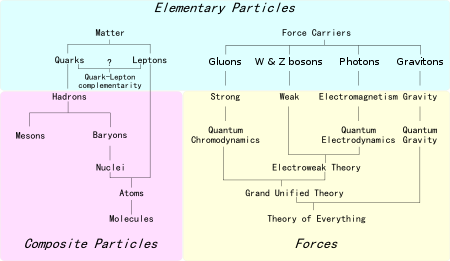• A proton
Proton
The proton is a subatomic particle with the symbol or and a positive electric charge of 1 elementary charge. One or more protons are present in the nucleus of each atom, along with neutrons. The number of protons in each atom is its atomic number....

and an electron
Electron
The electron is a subatomic particle with a negative elementary electric charge. It has no known components or substructure; in other words, it is generally thought to be an elementary particle. An electron has a mass that is approximately 1/1836 that of the proton...

can move separately; the total center-of-mass energy is positive, and such a pair of particles can be described as an ionized atom. Once the electron starts to "orbit" the proton, the energy becomes negative, and a bound state - namely the hydrogen atom
Hydrogen atom
A hydrogen atom is an atom of the chemical element hydrogen. The electrically neutral atom contains a single positively-charged proton and a single negatively-charged electron bound to the nucleus by the Coulomb force...

- is formed. Only the lowest energy bound state, the ground state
Ground state
The ground state of a quantum mechanical system is its lowest-energy state; the energy of the ground state is known as the zero-point energy of the system. An excited state is any state with energy greater than the ground state...

is stable. The other excited state
Excited state
Excitation is an elevation in energy level above an arbitrary baseline energy state. In physics there is a specific technical definition for energy level which is often associated with an atom being excited to an excited state....

s are unstable and will decay into bound states with less energy by emitting a photon
Photon
In physics, a photon is an elementary particle, the quantum of the electromagnetic interaction and the basic unit of light and all other forms of electromagnetic radiation. It is also the force carrier for the electromagnetic force...

.
• A nucleus
Atomic nucleus
The nucleus is the very dense region consisting of protons and neutrons at the center of an atom. It was discovered in 1911, as a result of Ernest Rutherford's interpretation of the famous 1909 Rutherford experiment performed by Hans Geiger and Ernest Marsden, under the direction of Rutherford. The...

is a bound state of proton
Proton
The proton is a subatomic particle with the symbol or and a positive electric charge of 1 elementary charge. One or more protons are present in the nucleus of each atom, along with neutrons. The number of protons in each atom is its atomic number....

s and neutron
Neutron
The neutron is a subatomic hadron particle which has the symbol or , no net electric charge and a mass slightly larger than that of a proton. With the exception of hydrogen, nuclei of atoms consist of protons and neutrons, which are therefore collectively referred to as nucleons. The number of...

s (nucleon
Nucleon
In physics, a nucleon is a collective name for two particles: the neutron and the proton. These are the two constituents of the atomic nucleus. Until the 1960s, the nucleons were thought to be elementary particles...

s).
• A positronium
Positronium
Positronium is a system consisting of an electron and its anti-particle, a positron, bound together into an "exotic atom". Being unstable, the two particles annihilate each other to produce two gamma ray photons after an average lifetime of 125 ps or three gamma ray photons after 142 ns in...

"atom" is an unstable bound state
Resonance
In physics, resonance is the tendency of a system to oscillate at a greater amplitude at some frequencies than at others. These are known as the system's resonant frequencies...

of an electron
Electron
The electron is a subatomic particle with a negative elementary electric charge. It has no known components or substructure; in other words, it is generally thought to be an elementary particle. An electron has a mass that is approximately 1/1836 that of the proton...

and a positron
Positron
The positron or antielectron is the antiparticle or the antimatter counterpart of the electron. The positron has an electric charge of +1e, a spin of ½, and has the same mass as an electron...

. It decays into photon
Photon
In physics, a photon is an elementary particle, the quantum of the electromagnetic interaction and the basic unit of light and all other forms of electromagnetic radiation. It is also the force carrier for the electromagnetic force...

s.
• The proton
Proton
The proton is a subatomic particle with the symbol or and a positive electric charge of 1 elementary charge. One or more protons are present in the nucleus of each atom, along with neutrons. The number of protons in each atom is its atomic number....

itself is a bound state of three quark
Quark
A quark is an elementary particle and a fundamental constituent of matter. Quarks combine to form composite particles called hadrons, the most stable of which are protons and neutrons, the components of atomic nuclei. Due to a phenomenon known as color confinement, quarks are never directly...

s (two up
Up quark
The up quark or u quark is the lightest of all quarks, a type of elementary particle, and a major constituent of matter. It, along with the down quark, forms the neutrons and protons of atomic nuclei...

and one down
Down quark
The down quark or d quark is the second-lightest of all quarks, a type of elementary particle, and a major constituent of matter. It, along with the up quark, forms the neutrons and protons of atomic nuclei...

; one red
Quantum chromodynamics
In theoretical physics, quantum chromodynamics is a theory of the strong interaction , a fundamental force describing the interactions of the quarks and gluons making up hadrons . It is the study of the SU Yang–Mills theory of color-charged fermions...

, one green
Quantum chromodynamics
In theoretical physics, quantum chromodynamics is a theory of the strong interaction , a fundamental force describing the interactions of the quarks and gluons making up hadrons . It is the study of the SU Yang–Mills theory of color-charged fermions...

and one blue
Quantum chromodynamics
In theoretical physics, quantum chromodynamics is a theory of the strong interaction , a fundamental force describing the interactions of the quarks and gluons making up hadrons . It is the study of the SU Yang–Mills theory of color-charged fermions...

). However, unlike the case of the hydrogen atom, the individual quarks can never be isolated. See confinement.

## In mathematical quantum physics

Let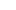be a complex separable Hilbert space,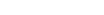be a one-parametric group of unitary operators onand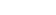be a statistical operator on. Let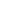be an observable onand let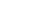be the induced probability distribution ofwith respect to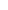on the Borel-algebra on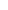. Then the evolution ofinduced by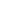is said to be bound with respect toif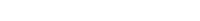, where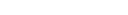.

Example:
Let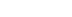and letbe the position observable. Let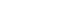have compact support and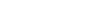.
• If the state evolution of"moves this wave package constantly to the right", e.g. if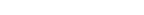for all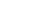, thenis not a bound state with respect to the position.

• Ifdoes not change in time, i.e.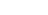for all, thenis a bound state with respect to position.

• More generally: If the state evolution of"just movesinside a bounded domain", thenis also a bound state with respect to position.

• Composite field
Composite field
In quantum field theory, a composite field is a field defined in terms of other more "elementary" fields. It might describe a composite particle or it might not. It might be local, or it might be nonlocal...

• Resonance
Resonance
In physics, resonance is the tendency of a system to oscillate at a greater amplitude at some frequencies than at others. These are known as the system's resonant frequencies...

• Bethe-Salpeter equation
Bethe-Salpeter equation
The Bethe–Salpeter equation, named after Hans Bethe and Edwin Salpeter, describes the bound states of a two-body quantum field theoretical system in a relativistically covariant formalism...